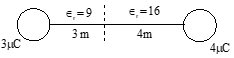Coulumbs law
Question

# The point charges  are placed at a separation of 7 m.  The medium between them is of two types as shown in figure, the electric force acting between them isDifficult
Solution

## For finding air equivalent separationWe have $\frac{1}{4\pi {ϵ}_{0}}\frac{{\mathrm{q}}_{1}{\mathrm{q}}_{2}}{{\mathrm{r}}^{\mathrm{\prime }2}}=\frac{1}{4\pi {\in }_{0}{\in }_{\mathrm{r}}}\frac{{\mathrm{q}}_{1}{\mathrm{q}}_{2}}{{\mathrm{r}}^{2}}$      ${\mathrm{F}}^{\mathrm{\prime }}=\frac{1}{4\pi {\in }_{0}}\frac{{\mathrm{q}}_{2}{\mathrm{q}}_{2}}{{\mathrm{r}}^{\mathrm{\prime }2}}$$⇒{\mathrm{r}}^{\mathrm{\prime }}=\sqrt{{ϵ}_{\mathrm{r}}}\mathrm{r}$       $=1.73×{10}^{-4}\mathrm{N}$Therefore , F' =

Get Instant Solutions